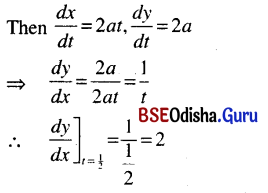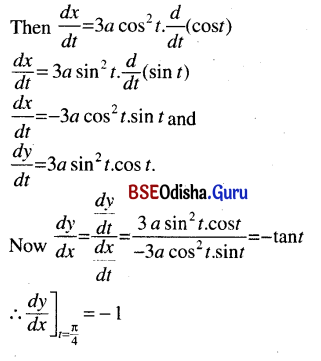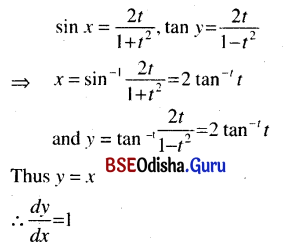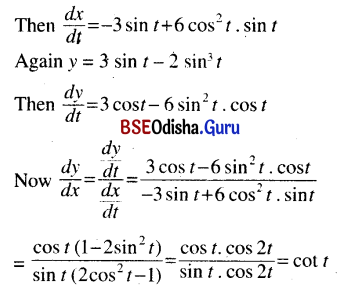# CHSE Odisha Class 12 Math Solutions Chapter 7 Continuity and Differentiability Ex 7(h)

Odisha State Board Elements of Mathematics Class 12 CHSE Odisha Solutions Chapter 7 Continuity and Differentiability Ex 7(h) Textbook Exercise Questions and Answers.

## CHSE Odisha Class 12 Math Solutions Chapter 7 Continuity and Differentiability Exercise 7(h)

Find $$\frac{d y}{d x}$$
Question 1.
x = a cos θ, y = a sin θ
Solution:
x = a cos θ, y = a sin θQuestion 2.
x = at2, y = 2 at at t = $$\frac{1}{2}$$
Solution:
x = at2, y = 2 at at t = $$\frac{1}{2}$$Question 3.
x = a cos3 t, y = a sin3 t at t = $$\frac{\pi}{4}$$
Solution:
x = a cos3 t, y = a sin3 tQuestion 4.
sin x = $$\frac{2 t}{1+t^2}$$, tan y = $$\frac{2 t}{1-t^2}$$
Solution:Question 5.
x = 3 cos t – 2 cos3 t,
y = 3 sin t – 2 sin3 t.
Solution:
x = 3 cos t – 2 cos3 t